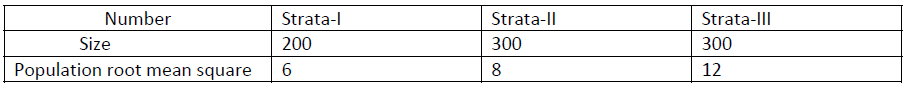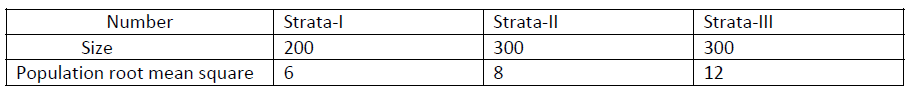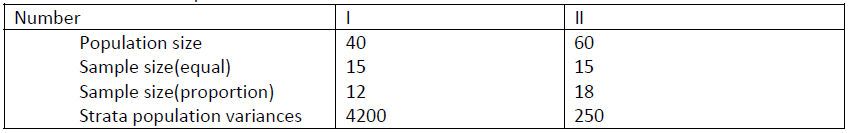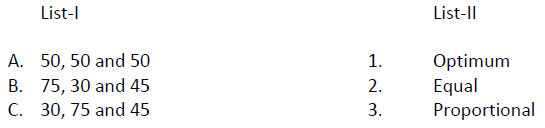51. The standard deviation of the marks obtained in mathematics by 109 students is found to be 12. The standard error of the estimator of the population mean for a random sample of size 9 using SRSWOR is:
(a) 3.58
(b) 3.45
(c) 3.85
(d) 4.00

52. For stratified random sampling, consider the following statements:
1. Units within each strata are as heterogeneous as possible
2. The strata mean are as homogeneous as possible

Which of the above statements is/are correct?
(a) 1 only
(b) 2 only
(c) Both 1 and 2
(d) Neither 1 nor 2

53. The theory of sampling is based on which of the following important principles?
1. Statistical regularity
2. Validity
3. Optimization

Select the correct answer using the code given below:
(a) 1 and 2 only
(b) 2 and 3 only
(c) 1 and 3 only
(d) 1, 2 and 3

54. Consider the following sampling procedures:
1. PPS
2. SRSWR
3. SRSWOR

In which of the above sampling procedures each member of the population gets a definite
probability of being included in the sample?
(a) 1 and 2 only
(b) 2 and 3 only
(c) 1 and 3 only
(d) 1, 2 and 3

55. A population of size 800 is divided into 3 strata. Following information is obtained:A stratified random sample of size 120 is to be drawn from the population. The sizes of the samples from the three strata under proportional allocation scheme are respectively:
(a) 30, 40, 50
(b) 30, 45, 45
(c) 20, 40, 60
(d) 50, 40, 30

56. A population of size 800 is divided into 3 strata. Following information is obtained:A stratified random sample of size 120 is to be drawn from the population. The sizes of the samples from the three strata under Neyman’s optimum allocation scheme are respectively:
(a) 30, 45, 45
(b) 20, 40, 60
(c) 20, 50, 50
(d) 20, 45, 55

57. Efficiency of the sample survey technique is measured by the inverse of the:
(a) Population variance
(b) Sample variance
(c) Sampling variance of the estimator
(d) Sample mean

58. Every unit of the population is enumerated in :
1. Sample survey
2. Census
3. Design of experiment

Which of the above is/are correct?
(a) 1 only
(b) 2 only
(c) 1 and 2 only
(d) 1, 2 and 3

59. The following information is obtained for a population of size 100 divided into two strata and a stratified random sample of size 30 was selected.For this data, consider the following statements:
1. The variance under equal allocation is more than that of proportional allocation
2. Stratified sampling with equal allocation is more precise than that of proportional allocation

Which of the statements is/are correct?
(a) 1 only
(b) 2 only
(c) Both 1 and 2
(d) Neither 1 nor 2